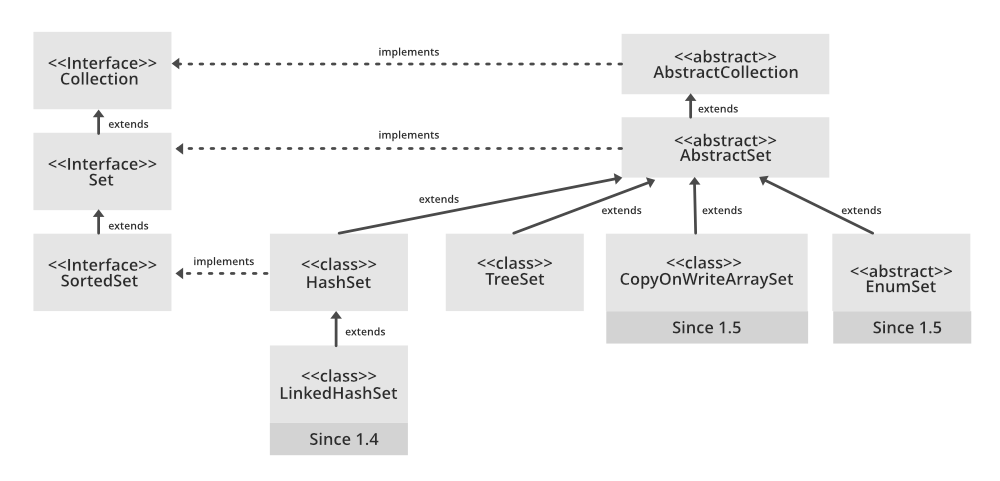GeeksforGeeks App
Open AppBrowser
Continue

# LinkedHashSet in Java with Examples

The LinkedHashSet is an ordered version of HashSet that maintains a doubly-linked List across all elements. When the iteration order is needed to be maintained this class is used. When iterating through a HashSet the order is unpredictable, while a LinkedHashSet lets us iterate through the elements in the order in which they were inserted. When cycling through LinkedHashSet using an iterator, the elements will be returned in the order in which they were inserted.

The Hierarchy of LinkedHashSet is as follows:Parameters: The type of elements maintained by this set

```All Implemented Interfaces are as listed below:
Serializable
Cloneable,
Iterable<E>
Collection<E>
Set<E>```

Syntax: Declaration

`public class LinkedHashSet<E> extends HashSet<E> implements Set<E>, Cloneable, Serializable`
• Contains unique elements only like HashSet. It extends the HashSet class and implements the Set interface.
• Maintains insertion order.

1. LinkedHashSet(): This constructor is used to create a default HashSet

`LinkedHashSet<E> hs = new LinkedHashSet<E>();`

2. LinkedHashSet(Collection C): Used in initializing the HashSet with the elements of the collection C.

`LinkedHashSet<E> hs = new LinkedHashSet<E>(Collection c);`

3. LinkedHashSet(int size): Used to initialize the size of the LinkedHashSet with the integer mentioned in the parameter.

`LinkedHashSet<E> hs = new LinkedHashSet<E>(int size);`

4. LinkedHashSet(int capacity, float fillRatio): Can be used to initialize both the capacity and the fill ratio, also called the load capacity of the LinkedHashSet with the arguments mentioned in the parameter. When the number of elements exceeds the capacity of the hash set is multiplied with the fill ratio thus expanding the capacity of the LinkedHashSet.

`LinkedHashSet<E> hs = new LinkedHashSet<E>(int capacity, int fillRatio);`

Example:

## Java

 `// Java Program to Illustrate LinkedHashSet` `// Importing required classes``import` `java.util.LinkedHashSet;` `// Main class``// LinkedHashSetExample``public` `class` `GFG {` `    ``// Main driver method``    ``public` `static` `void` `main(String[] args)``    ``{` `        ``// Creating an empty LinkedHashSet of string type``        ``LinkedHashSet linkedset``            ``= ``new` `LinkedHashSet();` `        ``// Adding element to LinkedHashSet``        ``// using add() method``        ``linkedset.add(``"A"``);``        ``linkedset.add(``"B"``);``        ``linkedset.add(``"C"``);``        ``linkedset.add(``"D"``);` `        ``// Note: This will not add new element``        ``// as A already exists``        ``linkedset.add(``"A"``);``        ``linkedset.add(``"E"``);` `        ``// Getting size of LinkedHashSet``        ``// using size() method``        ``System.out.println(``"Size of LinkedHashSet = "``                           ``+ linkedset.size());` `        ``System.out.println(``"Original LinkedHashSet:"``                           ``+ linkedset);` `        ``// Removing existing entry from above Set``        ``// using remove() method``        ``System.out.println(``"Removing D from LinkedHashSet: "``                           ``+ linkedset.remove(``"D"``));` `        ``// Removing existing entry from above Set``        ``// that does not exist in Set``        ``System.out.println(``            ``"Trying to Remove Z which is not "``            ``+ ``"present: "` `+ linkedset.remove(``"Z"``));` `        ``// Checking for element whether it is present inside``        ``// Set or not using contains() method``        ``System.out.println(``"Checking if A is present="``                           ``+ linkedset.contains(``"A"``));` `        ``// Noew lastly printing the updated LinkedHashMap``        ``System.out.println(``"Updated LinkedHashSet: "``                           ``+ linkedset);``    ``}``}`

Output

```Size of LinkedHashSet = 5
Original LinkedHashSet:[A, B, C, D, E]
Trying to Remove Z which is not present: false
Checking if A is present=true
Updated LinkedHashSet: [A, B, C, E]```

### Performing Various Operations on the LinkedHashSet Class

Let’s see how to perform a few frequently used operations on the LinkedHashSet.

In order to add an element to the LinkedHashSet, we can use the add() method. This is different from HashSet because in HashSet, the insertion order is not retained but is retained in the LinkedHashSet.

Example:

## Java

 `// Java Program to Add Elements to LinkedHashSet` `// Importing required classes``import` `java.io.*;``import` `java.util.*;` `// Main class``// AddingElementsToLinkedHashSet``class` `GFG {` `    ``// Main driver method``    ``public` `static` `void` `main(String[] args)``    ``{` `        ``// Creating an empty LinkedHashSet``        ``LinkedHashSet hs = ``new` `LinkedHashSet();` `        ``// Adding elements to above Set``        ``// using add() method` `        ``// Note: Insertion order is maintained``        ``hs.add(``"Geek"``);``        ``hs.add(``"For"``);``        ``hs.add(``"Geeks"``);` `        ``// Printing elements of Set``        ``System.out.println(``"LinkedHashSet : "` `+ hs);``    ``}``}`

Output:

`LinkedHashSet : [Geek, For, Geeks]`

Operation 2: Removing Elements

The values can be removed from the LinkedHashSet using the remove() method.

Example:

## Java

 `// Java program to Remove Elements from LinkedHashSet` `// Importing required classes``import` `java.io.*;``import` `java.util.*;` `// Main class``// RemoveElementsFromLinkedHashSet``class` `GFG {` `    ``// Main driver method``    ``public` `static` `void` `main(String[] args)``    ``{` `        ``// Creating an empty LinekdhashSet of string type``        ``LinkedHashSet hs``            ``= ``new` `LinkedHashSet();` `        ``// Adding elements to above Set``        ``// using add() method``        ``hs.add(``"Geek"``);``        ``hs.add(``"For"``);``        ``hs.add(``"Geeks"``);``        ``hs.add(``"A"``);``        ``hs.add(``"B"``);``        ``hs.add(``"Z"``);` `        ``// Printing all above elements to the console``        ``System.out.println(``"Initial HashSet "` `+ hs);` `        ``// Removing the element from above Set``        ``hs.remove(``"B"``);` `        ``// Again removing the element``        ``System.out.println(``"After removing element "` `+ hs);` `        ``// Returning false if the element is not present``        ``System.out.println(hs.remove(``"AC"``));``    ``}``}`

Output:

```Initial HashSet [Geek, For, Geeks, A, B, Z]
After removing element [Geek, For, Geeks, A, Z]
false```

Iterate through the elements of  LinkedHashSet using the iterator() method. The most famous one is to use the enhanced for loop.

Example:

## Java

 `// Java Program to Illustrate Iterating over LinkedHashSet` `// Importing required classes``import` `java.io.*;``import` `java.util.*;` `// Main class``// IteratingLinkedHashSet``class` `GFG {` `    ``// Main driver method``    ``public` `static` `void` `main(String[] args)``    ``{` `        ``// Instantiate an object of Set``        ``// Since LinkedHashSet implements Set``        ``// Set points to LinkedHashSet``        ``Set hs = ``new` `LinkedHashSet();` `        ``// Adding elements to above Set``        ``// using add() method``        ``hs.add(``"Geek"``);``        ``hs.add(``"For"``);``        ``hs.add(``"Geeks"``);``        ``hs.add(``"A"``);``        ``hs.add(``"B"``);``        ``hs.add(``"Z"``);` `        ``// Iterating though the LinkedHashSet``        ``// using iterators``        ``Iterator itr = hs.iterator();` `        ``while` `(itr.hasNext())``            ``System.out.print(itr.next() + ``", "``);` `        ``// New line``        ``System.out.println();` `        ``// Using enhanced for loop for iteration``        ``for` `(String s : hs)``            ``System.out.print(s + ``", "``);``        ``System.out.println();``    ``}``}`

Output:

```Geek, For, Geeks, A, B, Z,
Geek, For, Geeks, A, B, Z, ```

Here, E is the type of element stored.